# 前言• 原版高质量无水印图片下载太贵，由于没付费下载没有找到高质量图的高清无水印原图真实地址。没有办法(能力) 下载原版高清无水印。并且笔者也能猜测这个是一个网站的核心业务肯定也会层层设套。不会轻易获得,所以并没有对付费高清高质量无水印图片穷追不舍
• 但是高质量展示图在预览时候的是可以查看带有水印的高清图的(带着图虫创意水印)。
• 网站有一些免费的高清大图图片可以获取到。虽然这个不是精选图，但是质量也还可以

# 下载免费高清大图

https://stock.tuchong.com/topic?topicId=37 图虫创意url地址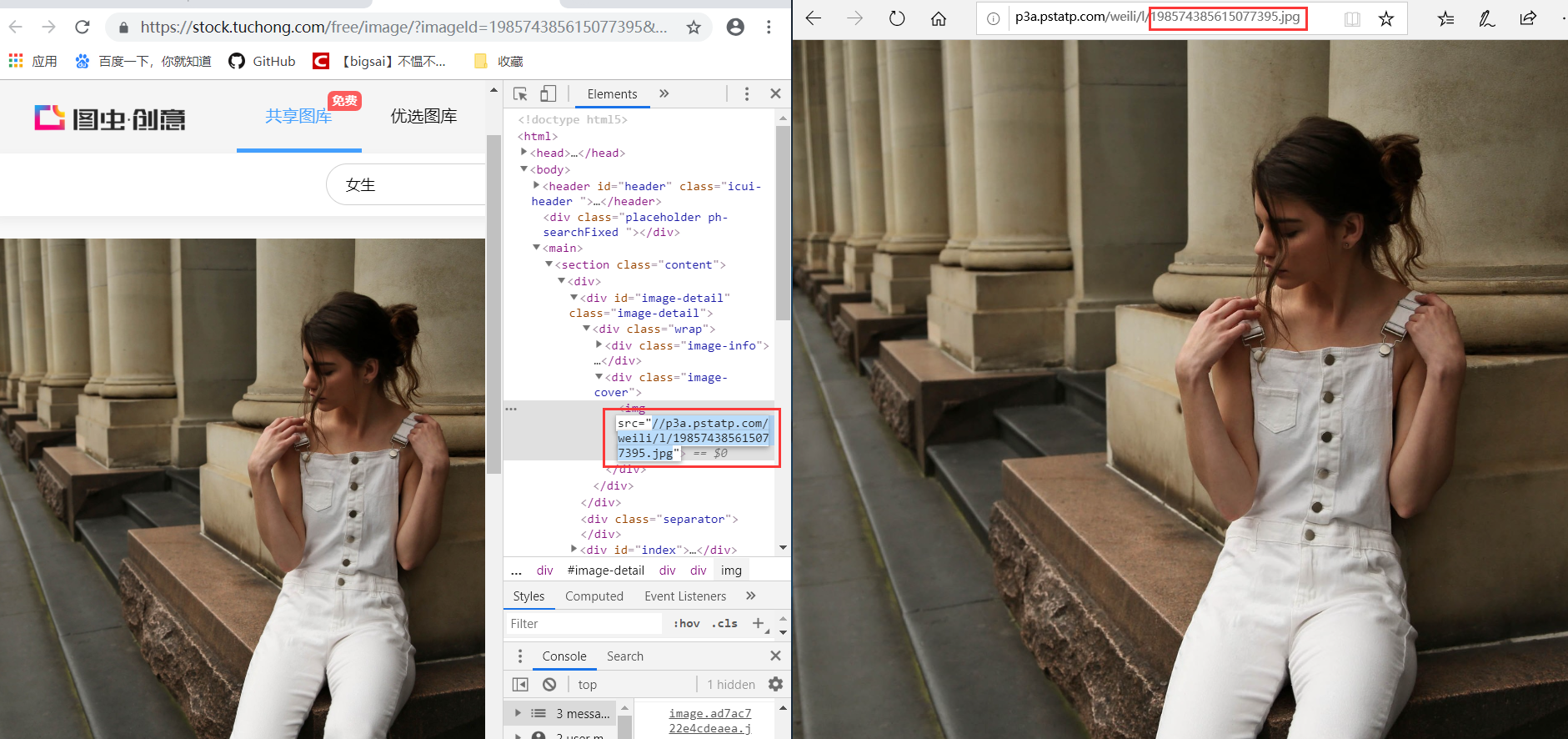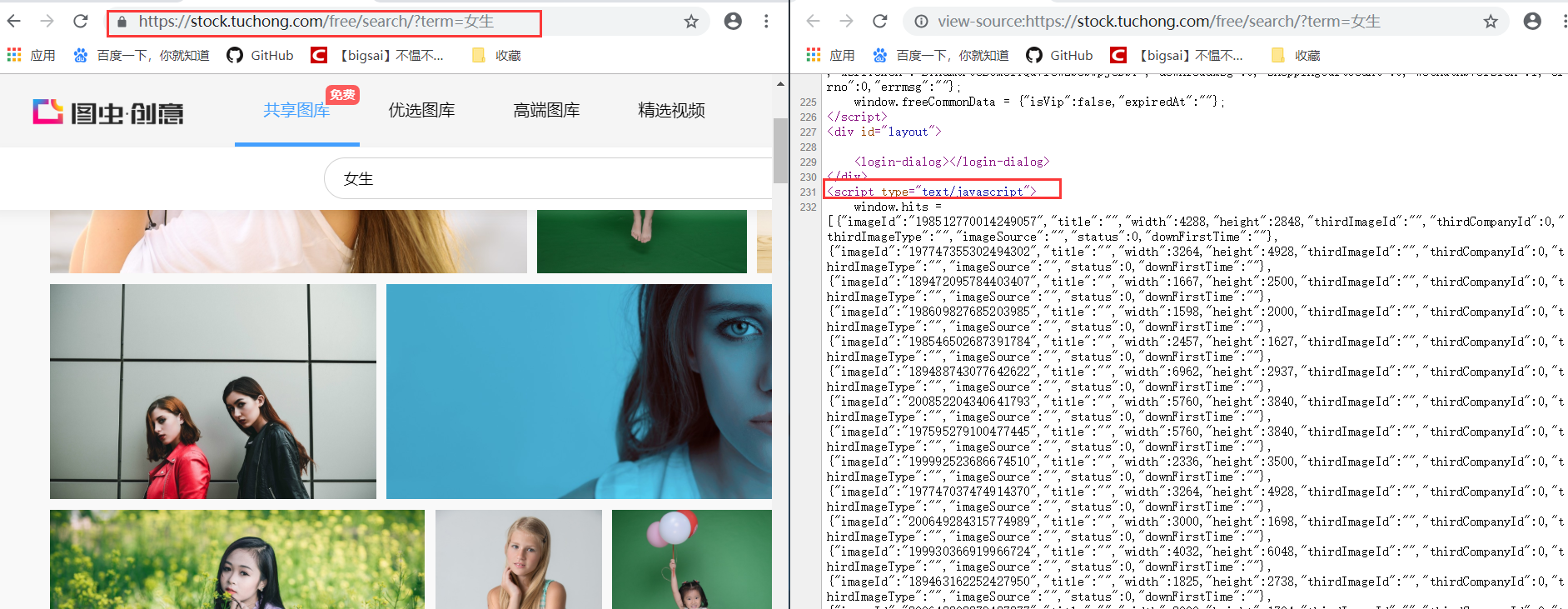# 下载带水印的精选图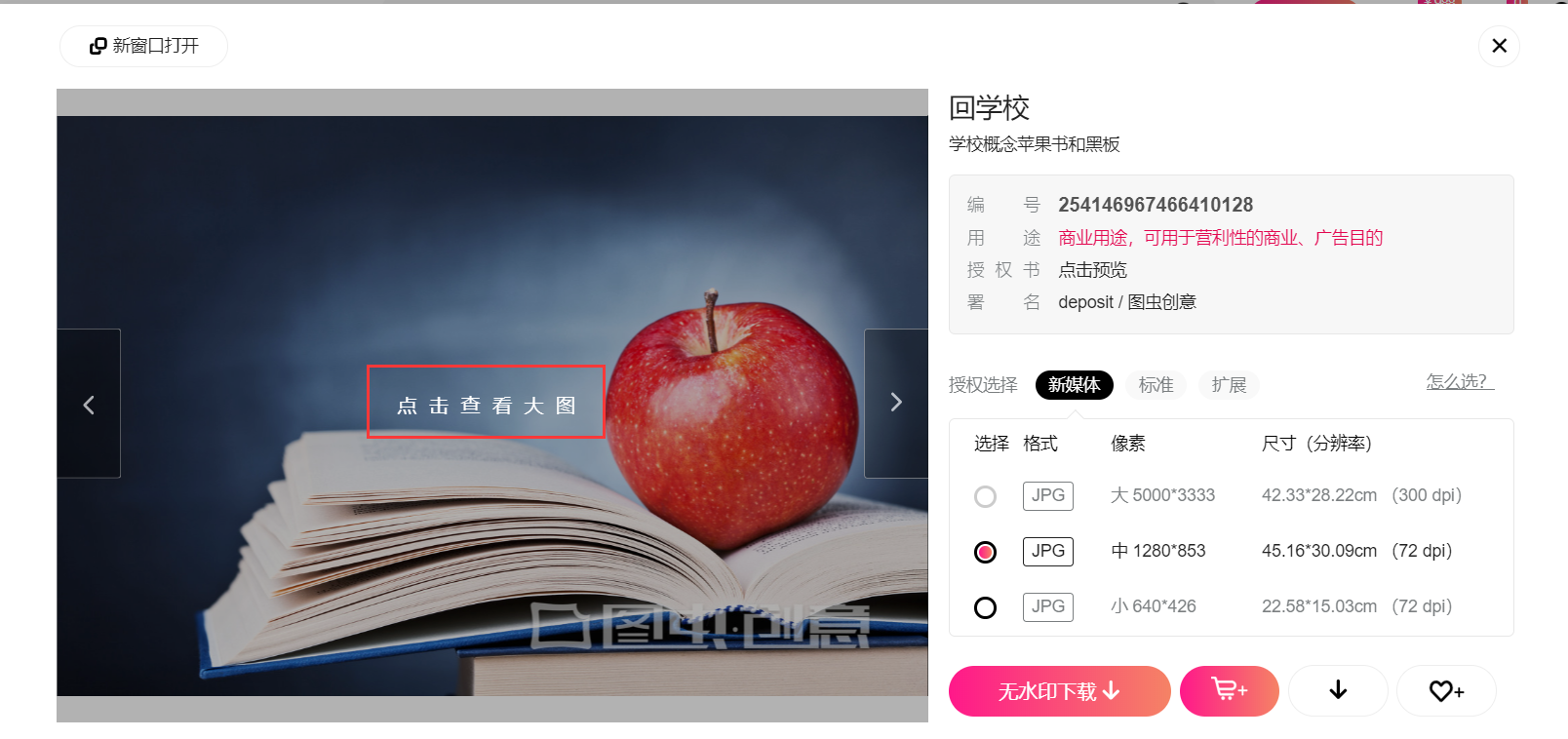##js的解析规则：
#----
js=soup.select('script') js=js
pattern = re.compile(r'window.hits = ($)(.*)($)')
va = pattern.search(str(js)).group(2)#解析js内容
#-------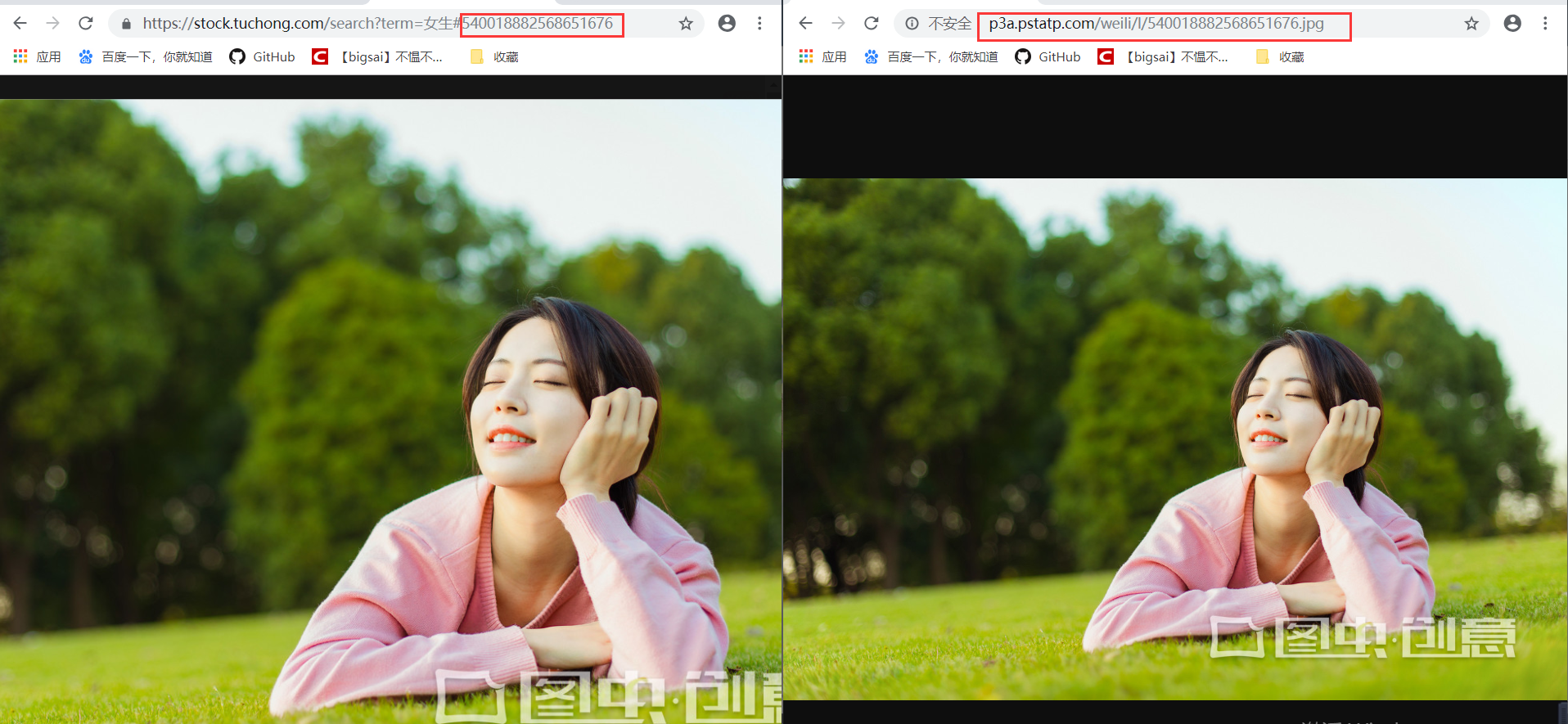# 代码与总结

import  requests
from  urllib import  parse
from bs4 import BeautifulSoup
import re
import json
'user-agent': 'Mozilla/5.0 (Windows NT 10.0; WOW64) AppleWebKit/537.36 (KHTML, like Gecko) Chrome/73.0.3683.86 Safari/537.36',
'Accept': 'text/html,application/xhtml+xml,application/xml;q=0.9,image/webp,image/apng,*/*;q=0.8,application/signed-exchange;v=b3',
'Accept-encoding': 'gzip, deflate, br',
'Accept-language': 'zh-CN,zh;q=0.9',
'Cache-Control': 'max-age=0',
'connection': 'keep-alive'
, 'Host': 'stock.tuchong.com',
}
def mkdir(path):
import os# 引入模块
path = path.strip()# 去除首位空格
path = path.rstrip("\\") # 去除尾部 \ 符号
isExists = os.path.exists(path)  # 判断路径是否存在  # 存在     True # 不存在   False
if not isExists:  # 判断结果
os.makedirs(path)# 如果不存在则创建目录 # 创建目录操作函数
return True#print (path + ' 创建成功')
else:
# 如果目录存在则不创建，并提示目录已存在
#print(path + ' 目录已存在')
return False
url = 'https://weiliicimg9.pstatp.com/weili/l/'+str(imageid)+'.webp'
url2 = 'https://icweiliimg9.pstatp.com/weili/l/'+str(imageid)+'.webp'
b=False
r = requests.get(url)
print(r.status_code)
if(r.status_code!=200):
r=requests.get(url2)
with open(imgname+'.jpg', 'wb') as f:
f.write(r.content)
print(imgname+" 下载成功")
def getText(text,free):
texturl = parse.quote(text)
url="https://stock.tuchong.com/"+free+"search?term="+texturl+"&use=0"
print(url)
soup=BeautifulSoup(req.text,'lxml')
js=soup.select('script')
path=''
if not free.__eq__(''):
js=js
path='无水印/'
else:
js=js
path='图虫创意/'
print(js)
pattern = re.compile(r'window.hits = ($)(.*)($)')
va = pattern.search(str(js)).group(2)#解析js内容
print(va)
va = va.replace('{', '{').replace('}', '},,')
print(va)
va = va.split(',,,')
print(va)
index = 1
for data in va:
try:
print(dict)
imgname='img2/'+path+text+'/'+dict['title']+str(index)
index+=1
mkdir('img2/'+path+text)
imgid=dict['imageId']
except Exception as e:
print(e)
if __name__ == '__main__':
num=input("高质量大图带水印输入1，普通不带水印输入2:")
num=int(num)
free=''
if num==2:
free='free/'
text = input('输入关键词:')
getText(text,free)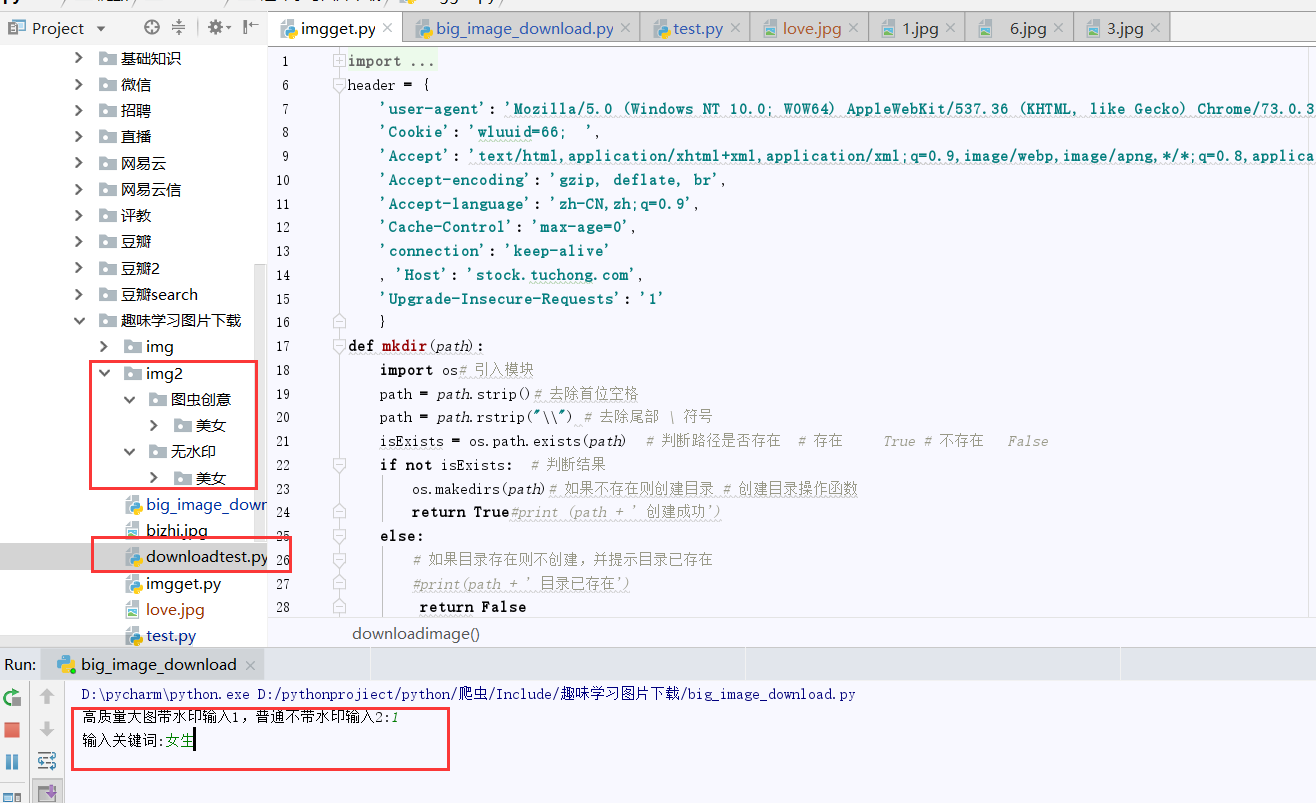IT圈不嫌多一个朋友，笔者也希望能成为你的朋友，共同学习，共同进步Big sai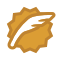CSDN认证博客专家 scikit-learn

03-19951807-252万+
10-181627
06-271132
07-114839